I.1.7a

12/21/20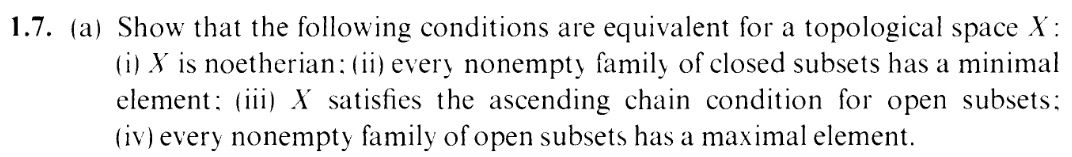Okay, I finally summoned the bravery to chip away at the 4-part 1.7. Well, at least part (a). And things actually proceeded quite smoothly... until I had an existential crisis, as you'll see.

We have to show that the following are equivalent:
(i) X is Noetherian (i.e. satisfies the desending chain condition on closed sets)
(ii) every nonempty family of closed subsets of X has a minimal element
(iii) X satisfies the ascending chain condition for open subsets
(iv) every nonempty family of open subsets of X has a maximal element

Taking a glance at it, it looks to me like
(i)(iii)(iv)(ii)(i) is the easiest order.

(i)(iii) is trivial. Just take complements.

I'll leave (iii)(iv) for last.

(iv)(ii) is also an easy "complements" argument

Let's show (ii)(i):

Given a descending chain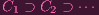(1)

of closed subsets of X.

Let L = {Ci}i=1. L has a minimal element Cn, by (ii). I.e. Cn CjCn = Cj. In particular, Cn = Cn+1 = Cn+2 =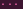, so we're done.

Okay, so everything is done except (iii)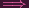(iv) (which is kind of the converse of (ii)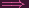(i), just the open set version).

Let L be a collection of open subsets of X. Suppose for the sake of contradiction that L does not have a maximal element. i.e.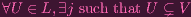(2)

Pick an arbitrary element V 1 L to begin a chain.
Suppose we already have a chain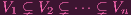(3)

Then using the maximality property, there is a V n+1 L such that V n V n+1. Hence we can add this to the chain: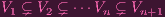(4)

Now, the handwavy thing to say would be, this process makes an infinite ascending proper chain of open subsets, contradicting (iii), and we'd be done.

Are you ready for some trippy stuff? Okay.

Something seems off with the reasoning to me. Do we really get an infinite chain? What I provided above was an inductive proof: For any natural number n, there is a chain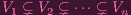(5)

I.e. I can construct chains of arbitrary finite length. I can make n as big as I want and extend the chain to that amount. But how does that translate to an infinite chain. Intuitively it seems like it would work, but I can't find a mathematical justification for it. And the ascending chain condition seems to always be stated in terms of infinite chains.

(Punchline: the "existential" crisis I was referring to at the start was regarding to the existence of the infinite chain, which is nevertheless more troubling than screaming "WHY DO I EX1ST!?!?111?!!?" to myself)

I understand that I am making infinitely many choices, but what I'm doing here seems to require MORE than just using the axiom of Choice. The axiom of Choice allows me to pick one element per a collection of sets, right? But for Choice, that collection is presumably predetermined. The choices I'm making here aren't from a predetermined collection of sets, because in my proof, each choice DEPENDS ON THE LAST CHOICE. That seems worse than pure Choice.

So the question remains: How finite chains of arbitrary length extend to an infinite chain, especially when each finite chain depends on the last?

UPDATE: I just looked up several proofs, and they're pretty much verbatim to mine. I.e., they just assume that an infinite chain exists by the inductive logic. One of them mentions Choice, but like I said, that doesn't seem sufficient. I'm not convinced. I know it seems intuitive, but I can't wrap my head around it.

UPDATE: Okay, after like an hour or two of thinking about it, in desperation, I literally looked up "axiom of dependent choice", and it exists. This precisely solves my problem. I was asking, "how does an arbitrary finite length chain, where the choice of each chain depedns on the last, extend to an infinite length chain?" And, as it states,

Even without such an axiom, for any n, one can use ordinary mathematical induction to form the first n terms of such a sequence. The axiom of dependent choice says that we can form a whole (countably infinite) sequence this way.

There we go. So dependent finite length chains extend to infinite length chains, if you accept this axiom.

However, surprisingly, according to that page, AC ("full" Choice) implies DC (dependent choice). I thought AC wasn't enough alone, but it is. It implies the very thing I need.

This begs the question: How is AC stronger than DC, when it seems like for AC, the sets you're picking from are already determined? Check this out:

"Axiom of Choice Implies Axiom of Dependent Choice"

Holy fuck, that is trippy. But it makes sense.

Following that proof, the way it works for the "infinite chain" example above is that you use induction to show that every R(a) is nonempty i.e. to show that every U L has a "successor" element V U, and THEN use Choice to pick a single successor for each element, and finally pick any element and construct a chain using the now prepicked successors.

That... feels nontrivial to me. No wonder Choice is so controversial.